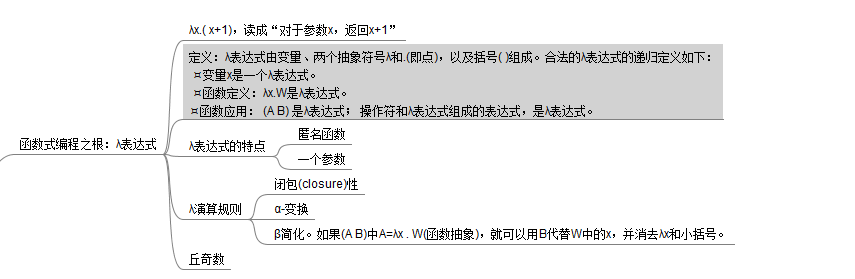# 函数式编程之根-拉姆达运算/演算(λ-calculus)

0.1.6函数编程范式 】

1930s初，普林斯顿大学的逻辑学家阿伦佐·丘奇 (Alonzo Church，1903-1995) 开发出了一种新的形式系统(formal system)，即拉姆达运算/演算 (λ-calculus 、lambda calculus ，lambda即希腊字母λ)。由于任何一个可计算函数都能用λ演算来表达和求值，它等价于图灵机。λ演算决定或形成了函数式编程范式。函数式语言的典型代表有Lisp、Scheme、ML、Haskell和Erlang等等，λ演算是函数式编程语言共同的祖先。

★函数编程范式的核心观念：以λ表达式构造一切。

## 什么是λ表达式呢？

λ表达式极其简洁，由变量、操作符(假定被定义)、两个抽象符号λ和.(即点)，以及括号( )组成。合法的λ表达式的递归定义如下：

• 变量(和文字)是一个λ表达式。
• 操作符和λ表达式组成的表达式，是λ表达式。(可以纳入函数应用)
• 函数定义和函数应用是λ表达式。

C#语言：(x) =>{ return x+1; }

Java语言：(x) ->{ return x+1; }

Scheme语言：(lambda (x) (+ x 1))

## 1.“归根结底”的含义

``````;;;例程 0-4 核心观念远离程序员
;;; 归根结底.rkt
(define T  (lambda (x) (lambda (y) x))   ) ;;; true
((T 1) 2) ;;; 输出1.
(define F  (lambda (x) (lambda (y) y))   );;; false
(define And  (lambda (x) (lambda (y) (x y) F)   ))
((And T) F ) ;;;#<procedure:f> (> 3 2) ;;; 输出 #t,这才是程序员需要的
``````

• 数据的不变性。
• 使用递归作为循环的机制。
• 函数没有副作用和引用透明。参考[编程导论(Java )·3.1.2 方法]
• 支持高阶函数(higher-order functions) 和闭包(closures)。参考[2.3高阶函数]
• 支持惰性计算(lazy evaluation)。

2.学习函数式编程，从了解变量开始

3. 函数定义和函数应用

•  函数抽象：W是参数为变量x的λ表达式，则λx.W是λ表达式。这种表达式给出了一个函数的定义：W是函数体，形参就是变量x。
λx.( x+1)
λx. λy. ( x+y) ;;;表示 λx. (λy. ( x+y))
•  函数应用：有了f(x)=x+1，自然需要计算f(2)，即给函数一个(实际)参数进行求值。A、B是λ表达式，则 (A B) 也是λ表达式，表示将实参B带入函数A中。### 2.函数定义的特点

• 匿名函数。数学函数如f(x)=x+1或λ表达式如λx.( x+1)，描述了一个计算过程；数学函数的f是函数名，而λ表达式关注计算过程，为该函数命名，变成了程序员的事情。
• 每个函数只有一个输入参数，如λn. λm.λf.λx. ((n f)  (++ m) )。编程时函数通常可以有一个参数列表，逻辑学家 Haskell Curry证明可以将一个拥有多个参数的函数转化为只有一个参数的多个函数的连续调用，这一转化过程称为对拥有多个参数的函数的currying/柯里化
3.通过 丘奇数，熟悉 λ演算的α-变换和β简化。